# Exploring The Quick, Draw! Dataset With R: The Mona Lisa

All that noise, and all that sound, all those places I have found (Speed of Sound, Coldplay)

Some days ago, my friend Jorge showed me one of the coolest datasets I’ve ever seen: the Google quick draw dataset. In its Github website you can see a detailed description of the data. Briefly, it contains  around 50 million of drawings of people around the world in `.ndjson` format. In this experiment, I used the simplified version of drawings where strokes are simplified and resampled with a 1 pixel spacing. Drawings are also aligned to top-left corner and scaled to have a maximum value of 255. All these things make data easier to manage and to represent into a plot.

Since `.ndjson` files may be very large, I used `LaF` package to access randon lines of the file rather than reading it completely. I wrote a script to explore `The Mona Lisa.ndjson` file, which contains more than 120.000 drawings that the TensorFlow engine from Google recognized as being The Mona Lisa. It is quite funny to see them. Whit this script you can:

• Reproduce a random single drawing
• Create a 9×9 mosaic of random drawings
• Create an animation simulating the way the drawing was created

I use ggplot2 package to render drawings and `gganimate` package of David Robinson to create animations.

This is an example of a single drawing:This is an example of a 3×3 mosaic:This is an example of animation:If you want to try by yourself, you can find the code here.

Note: to work with `gganimate`, I downloaded the portable version and pointed to it with `Sys.setenv` command as explained here.

# Coloring Sudokus

Someday you will find me
caught beneath the landslide
(Champagne Supernova, Oasis)

I recently read a book called Snowflake Seashell Star: Colouring Adventures in Numberland by Alex Bellos and Edmund Harris which is full of mathematical patterns to be coloured. All images are truly appealing and cause attraction to anyone who look at them, independently of their age, gender, education or political orientation. This book demonstrates how maths are an astonishing way to reach beauty.

One of my favourite patterns are tridokus, a sophisticated colored version of sudokus. Coloring a sudoku is simple: once that is solved it is enough to assign a color to each number (from 1 to 9).  If you superimpose three colored sudokus with no cells at the same position sharing the same color, and using again nine colors, the resulting image is a tridoku: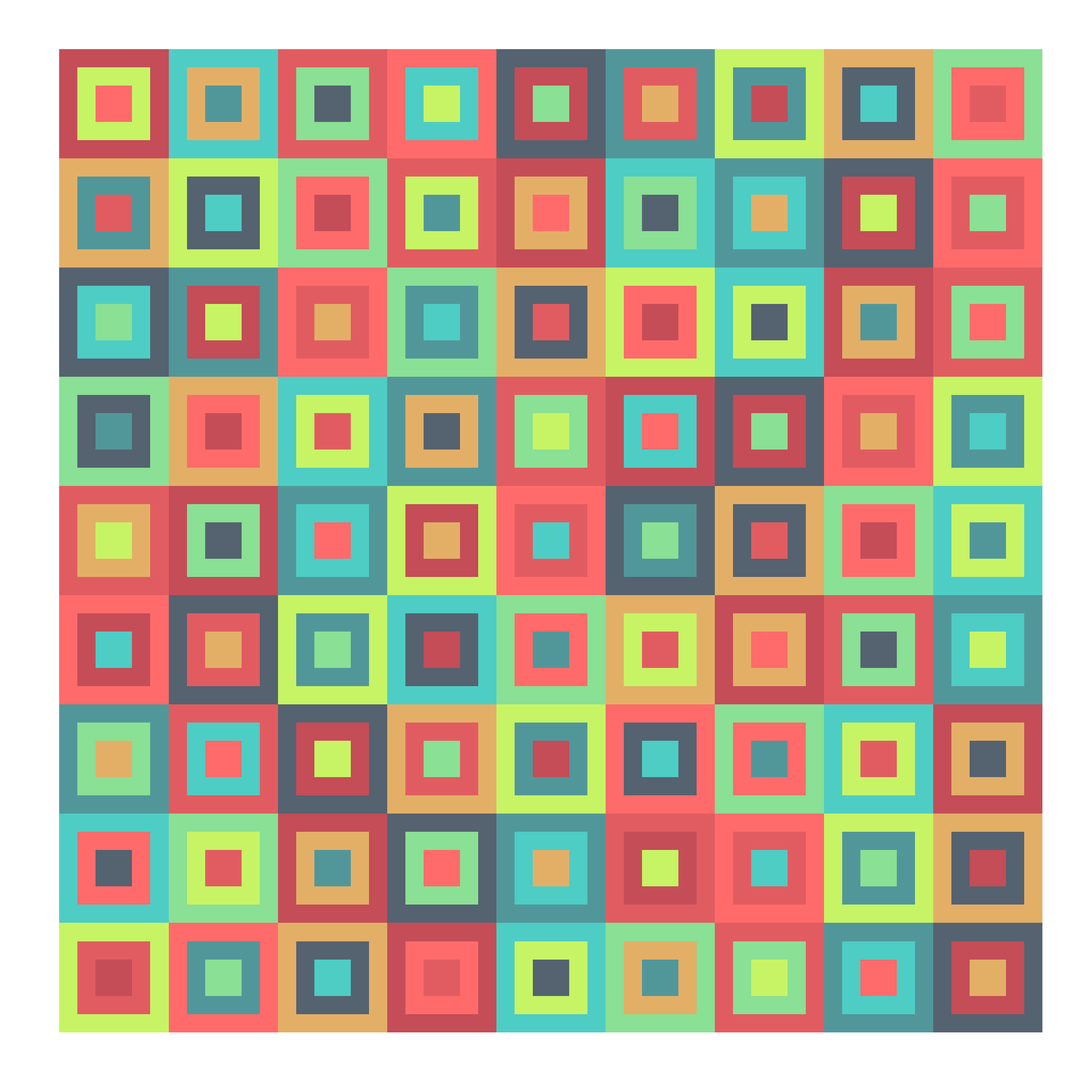There is something attractive in a tridoku due to the balance of colors but also they seem a quite messy: they are a charmingly unbalanced.  I wrote a script to generalize the concept to n-dokus. The idea is the same: superimpose n sudokus without cells sharing color and position (I call them disjoint sudokus) using just nine different colors. I did’n’t prove it, but I think the maximum amount of sudokus can be overimposed with these constrains is 9. This is a complete series from 1-doku to 9-doku (click on any image to enlarge):

I am a big fan of `colourlovers` package. These tridokus are colored with some of my favourite palettes from there:

Just two technical things to highlight:

• There is a package called sudoku that generates sudokus (of course!). I use it to obtain the first solved sudoku which forms the base.
• Subsequent sudokus are obtained from this one doing two operations: interchanging groups of columns first (there are three groups: columns 1 to 3, 4 to 6 and 7 to 9) and interchanging columns within each group then.

You can find the code here: do you own colored n-dokus!

# Pencil Scribbles

Con las bombas que tiran los fanfarrones, se hacen las gaditanas tirabuzones (Palma y corona, Carmen Linares)

This time I draw Franky again using an algorithm to solve the Travelling Salesman Problem as I did in my last post. On this occasion, instead of doing just one single line drawing, I overlap many of them (250 concretely), each of them sampling 400 points on the original image (in my previous post I sampled 8.000 points). Last difference is that I don’t convert the image to pure black and white with `threshold` function: now I use the gray scale number of each pixel to weight the sample.

Once again, I use `ggplot2` package, and its magical `geom_path`, to generate the image. The pencil effect is obtained giving a very high transparency to the lines. This is the result:I love when someone else experiment with my experiments as Mara Averick did:

You can do it as well with this one, since you will find the code here. Please, let me know your own creations if you do. You can find me on twitter or by email.

P.S.: Although it may seems otherwise, I’m not obsessed with Frankenstein 🙂

# The Travelling Salesman Portrait

I have noticed even people who claim everything is predestined, and that we can do nothing to change it, look before they cross the road (Stephen Hawking)

Imagine a salesman and a set of cities. The salesman has to visit each one of the cities starting from a certain one and returning to the same city. The challenge is finding the route which minimizes the total length of the trip. This is the Travelling Salesman Problem (TSP): one of the most profoundly studied questions in computational mathematics. Since you can find a huge amount of articles about the TSP in the Internet, I will not give more details about it here.

In this experiment I apply an heuristic algorithm to solve the TSP to draw a portrait. The idea is pretty simple:

• Convert it to black and white
• Choose a sample of black points
• Solve the TSP to calculate a route among the points
• Plot the route

The result is a single line drawing of the image that you loaded. To solve the TSP I used the arbitrary insertion heuristic algorithm (Rosenkrantz et al. 1977), which is quite efficient.

To illustrate the idea, I have used again this image of Frankenstein (I used it before in this other experiment). This is the result:You can find the code here.

# Mandalas Colored

Apriétame bien la mano, que un lucero se me escapa entre los dedos (Coda Flamenca, Extremoduro)

I have the privilege of being teacher at ESTALMAT, a project run by Spanish Royal Academy of Sciences that tries to detect, guide and stimulate in a continuous way, along two courses, the exceptional mathematical talent of students of 12-13 years old. Some weeks ago I gave a class there about the importance of programming. I tried to convince them that learning R or Python is a good investment that always pays off; It will make them enjoy more of mathematics as well as to see things with their own eyes. The main part of my class was a workshop about Voronoi tesselations in R. We started drawing points on a circle and we finished drawing mandalas like these ones. You can find the details of the workshop here (in Spanish). It was a wonderful experience to see the faces of the students while generating their own mandalas.

In that case all mandalas were empty, ready to be printed and coloured as my 7 years old daughter does. In this experiment I colour them. These are the changes I have done to my  previous code:

• Remove external segments which intersects the boundary of the enclosing
rectangle
• Convert the tesselation into a list of polygons with `tile.list` function
• Use `colourlovers` package to fill the polygons with beautiful colour palettes

This is an example of the result:Changing three simple parameters (`iter`, `points` and `radius`) you can obtain completely different images (clicking on any image you can see its full size version):

You can find details of these parameters in my previous post. I cannot resist to place more examples:

You can find the code here. Enjoy.

# Mandalas

Mathematics is a place where you can do things which you can’t do in the real world (Marcus Du Sautoy, mathematician)

From time to time I have a look to some of my previous posts: it’s like seeing them through another’s eyes. One of my first posts was this one, where I draw fractals using the Multiple Reduction Copy Machine (MRCM) algorithm. That time I was not clever enough to write an efficient code able generate deep fractals. Now I am pretty sure I could do it using `ggplot` and I started to do it when I come across with the idea of mixing this kind of fractal patterns with Voronoi tessellations, that I have explored in some of my previous posts, like this one. Mixing both techniques, the mandalas appeared.

I will not explain in depth the mathematics behind this patterns. I will just give a brief explanation:

• I start obtaining `n` equidistant points in a unit circle centered in `(0,0)`
• I repeat the process with all these points, obtaining again `n` points around each of them; the radius is scaled by a factor
• I discard the previous (parent) `n` points

I repeat these steps iteratively. If I start with n points and iterate k times, at the end I obtain nk points. After that, I calculate the Voronoi tesselation of them, which I represent with `ggplot`.

This is an example:Some others: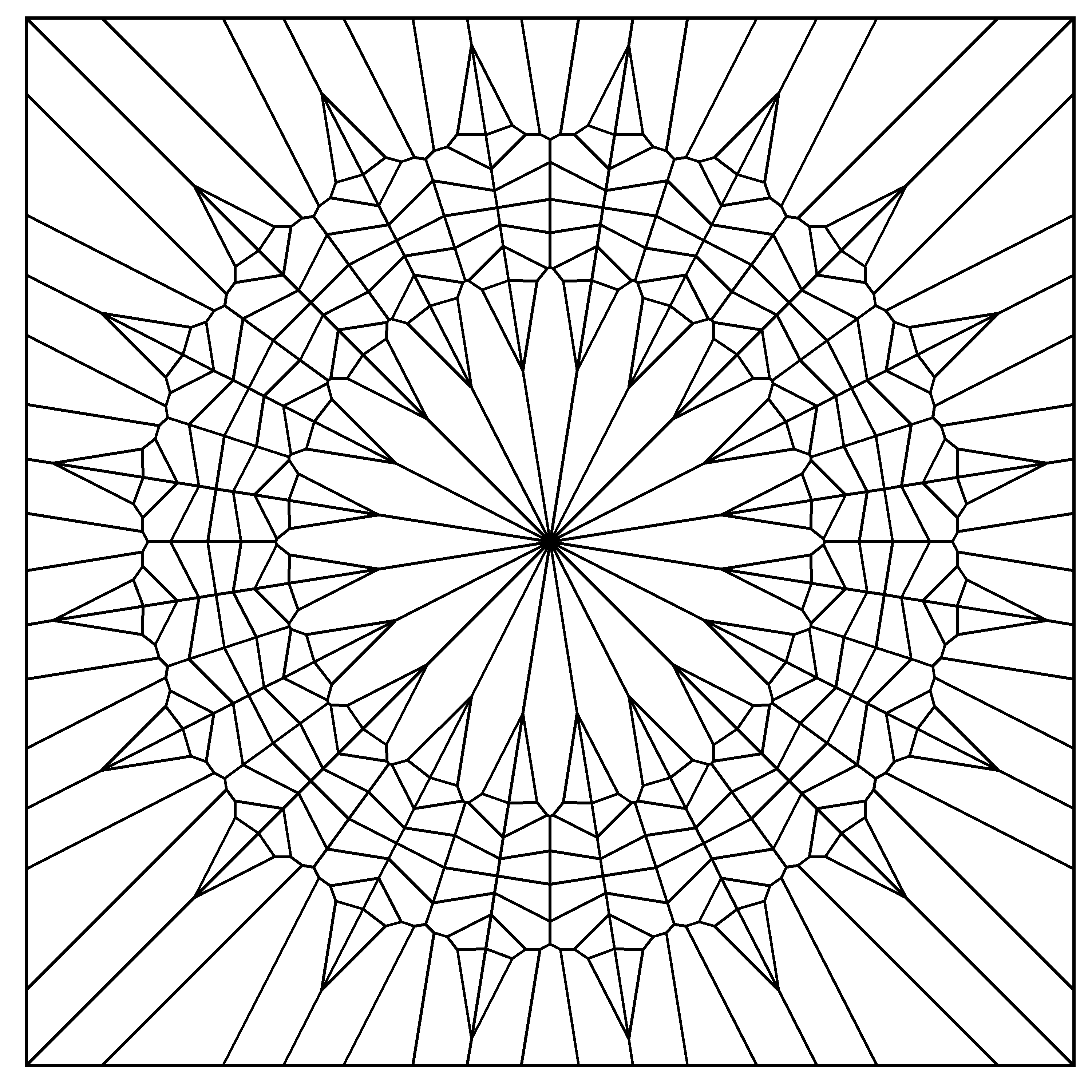You can find the code here. Enjoy it.

# Tiny Art in Less Than 280 Characters

Now that Twitter allows 280 characters, the code of some drawings I have made can fit in a tweet. In this post I have compiled a few of them.

The first one is a cardioid inspired in string art (more info here):

```library(ggplot2)
n=300
t1=1:n
t0=seq(3,2*n+1,2)%%n
t2=t0+(t0==0)*n
df=data.frame(x=cos((t1-1)*2*pi/n),
y=sin((t1-1)*2*pi/n),
x2=cos((t2-1)*2*pi/n),
y2=sin((t2-1)*2*pi/n))
ggplot(df,aes(x,y,xend=x2,yend=y2)) +
geom_segment(alpha=.1)+theme_void()
``````library(ggplot2)
library(dplyr)
t=seq(from=0, to=100*pi, length.out=500*100)
data.frame(x= t^(1/2)*cos(t), y= t^(1/2)*sin(t))%>%
rbind(-.)%>%ggplot(aes(x, y))+geom_polygon()+theme_void()
```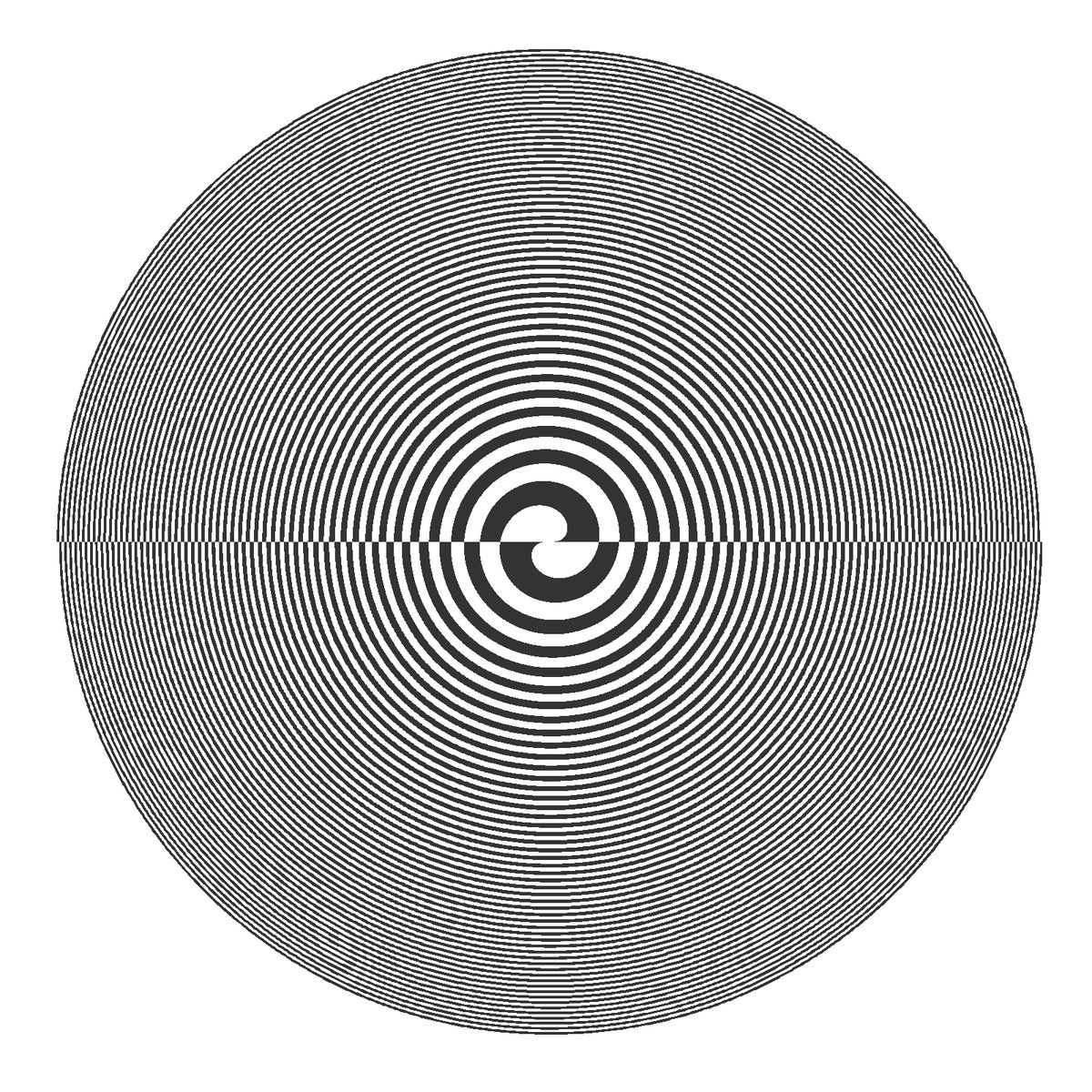```library(dplyr)
library(ggplot2)
library(pracma)
seq(-5*pi,5*pi,by=.1)%>%expand.grid(x=., y=.)%>%
ggplot(aes(x=x, y=y, fill=erf(sec(x)-sec(y))))+geom_tile()+
theme_void()+theme(legend.position="none")
```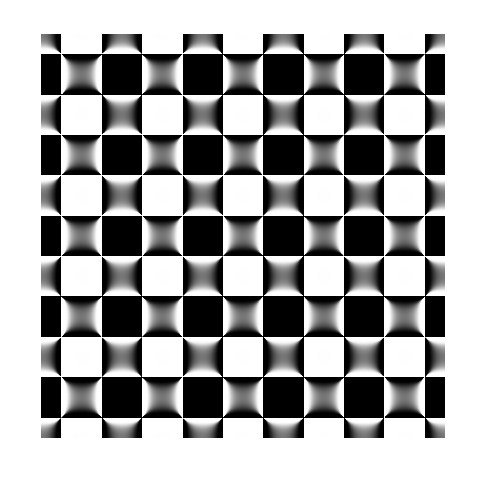A x-y scatter plot of a trigonometric function on R2 (more info here):

```library(dplyr)
library(ggplot2)
seq(from=-10, to=10, by = 0.05) %>%
expand.grid(x=., y=.) %>%
ggplot(aes(x=(x+pi*sin(y)), y=(y+pi*sin(x)))) +
geom_point(alpha=.1, shape=20, size=1, color="black")+
theme_void()
``````library(TurtleGraphics)
turtle_init()
turtle_col("gray25")
turtle_do({
for (i in 1:150) {
turtle_forward(dist=1+0.5*i)
turtle_right(angle=89.5)}
})
turtle_hide()
```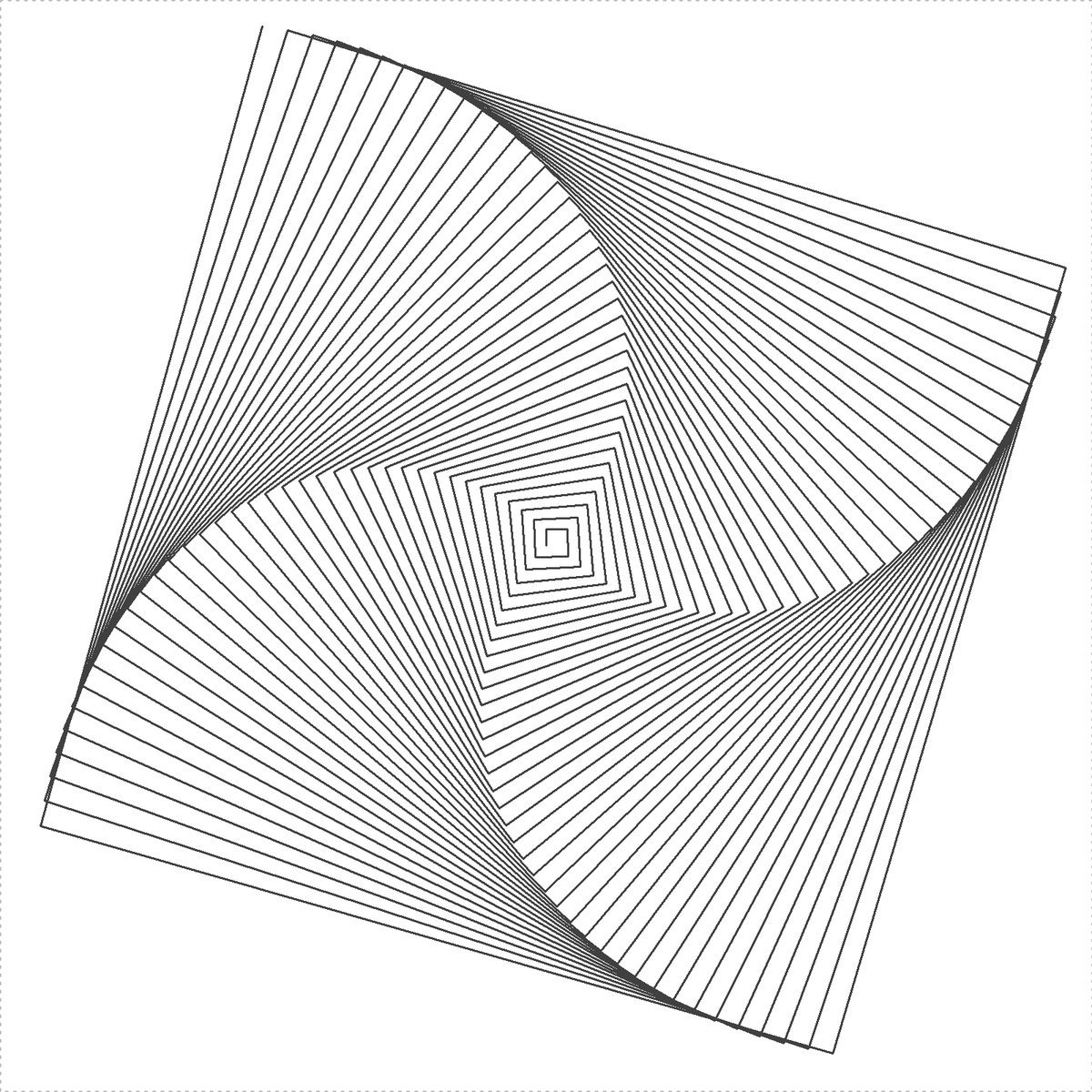```t=seq(1, 100, by=.001)
plot(exp(-0.006*t)*sin(t*3.019+2.677)+
exp(-0.001*t)*sin(t*2.959+2.719),
exp(-0.009*t)*sin(t*2.964+0.229)+
exp(-0.008*t)*sin(t*2.984+1.284),
type="l", axes=FALSE, xlab="", ylab="")
``````library(circlize)
chordDiagram(matrix(1, 20, 20), symmetric = TRUE,
col="black", transparency = 0.85, annotationTrack = NULL)
```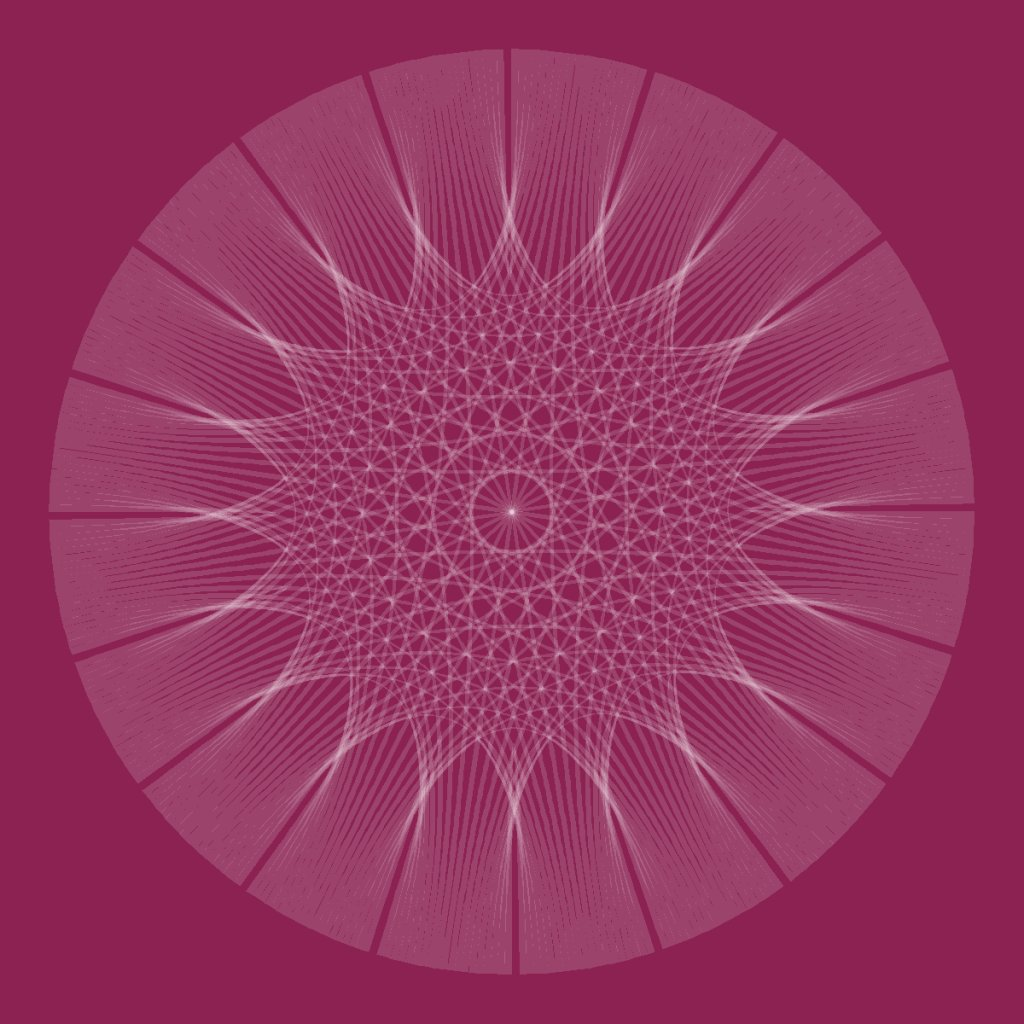Most of them are made with `ggplot2` package. I love R and the sense of wonder of how just one or two lines of code can create beautiful and unexpected patterns.

I recently did this project for DataCamp to show how easy is to do art with R and `ggplot`. Starting from a extremely simple plot, and following a well guided path, you can end making beautiful images like this one:Furthermore, you can learn also `ggplot2` while you do art.

I have done the project together with Rasmus Bååth, instructor at DataCamp and the perfect mate to work with. He is looking for people to build more projects so if you are interested, here you can find more information. Do not hesitate to ask him for details.

All the best for 2018.

Merry Christmas.

# Drawing 10 Million Points With ggplot: Clifford Attractors

For me, mathematics cultivates a perpetual state of wonder about the nature of mind, the limits of thoughts, and our place in this vast cosmos (Clifford A. Pickover – The Math Book: From Pythagoras to the 57th Dimension, 250 Milestones in the History of Mathematics)

I am a big fan of Clifford Pickover and I find inspiration in his books very often. Thanks to him, I discovered the harmonograph and the Parrondo’s paradox, among many other mathematical treasures. Apart of being a great teacher, he also invented a family of strange attractors wearing his name. Clifford attractors are defined by these equations:$x_{n+1}\, =\, sin(a\, y_{n})\, +\, c\, cos(a\, x_{n}) \\ y_{n+1}\, =\, sin(b\, x_{n})\, +\, d\, cos(b\, y_{n}) \\$

There are infinite attractors, since a, b, c and d are parameters. Given four values (one for each parameter) and a starting point `(x0, y0)`, the previous equation defines the exact location of the point at step `n`, which is defined just by its location at `n-1`; an attractor can be thought as the trajectory described by a particle. This plot shows the evolution of a particle starting at `(x0, y0)=(0, 0)` with parameters `a=-1.24458046630025`, `b=-1.25191834103316`, `c=-1.81590817030519` and `d=-1.90866735205054` along 10 million of steps:Changing parameters is really entertaining. Drawings have a sandy appearance: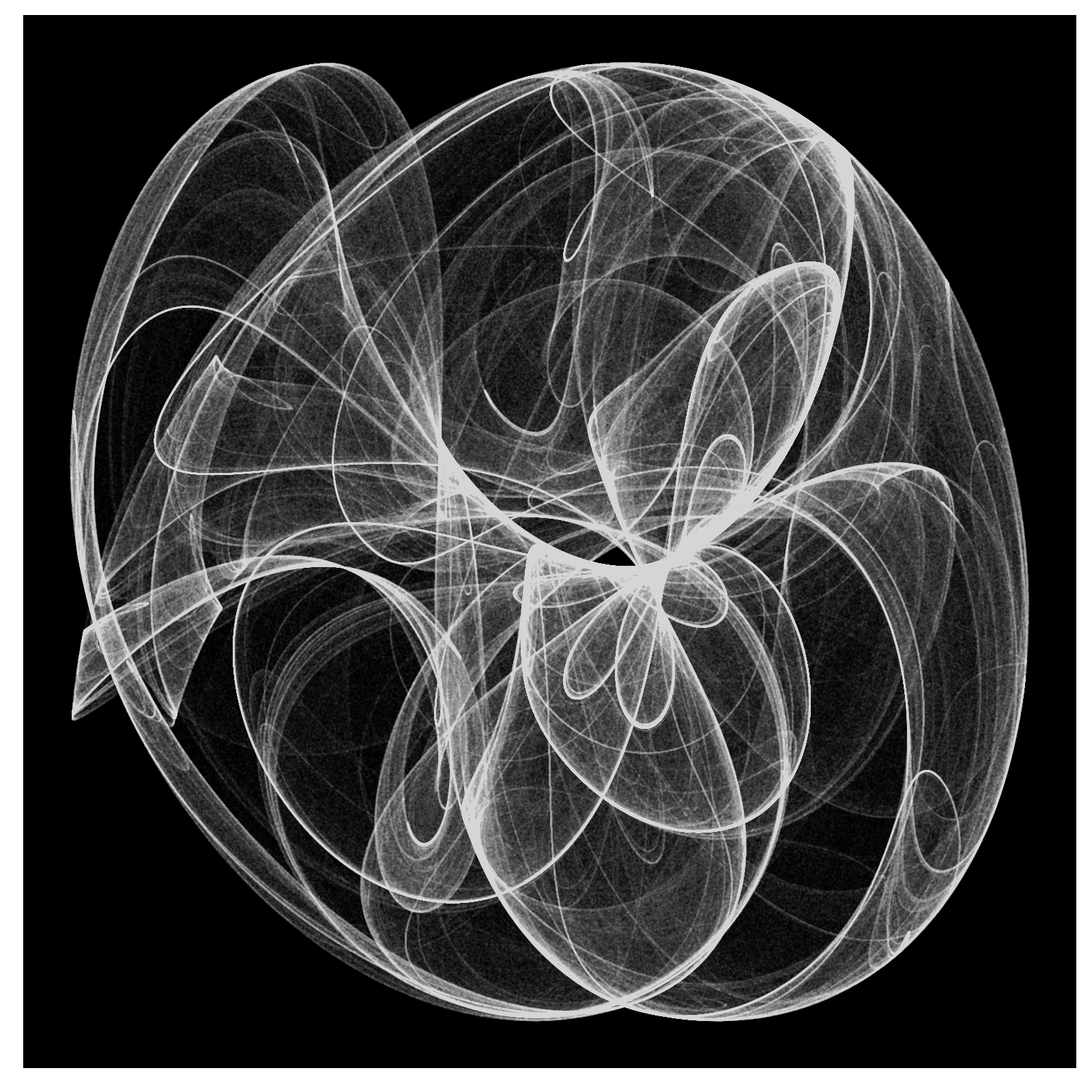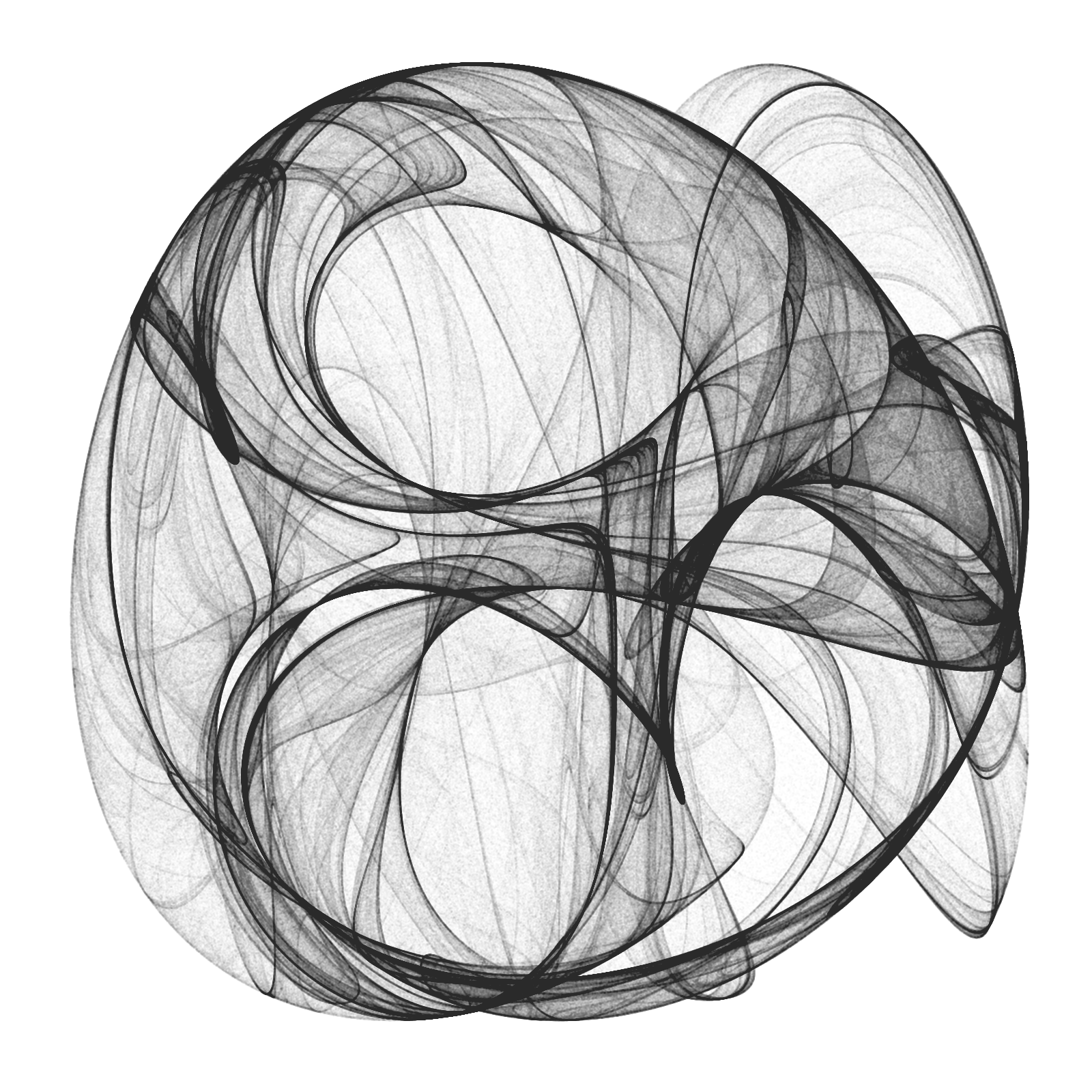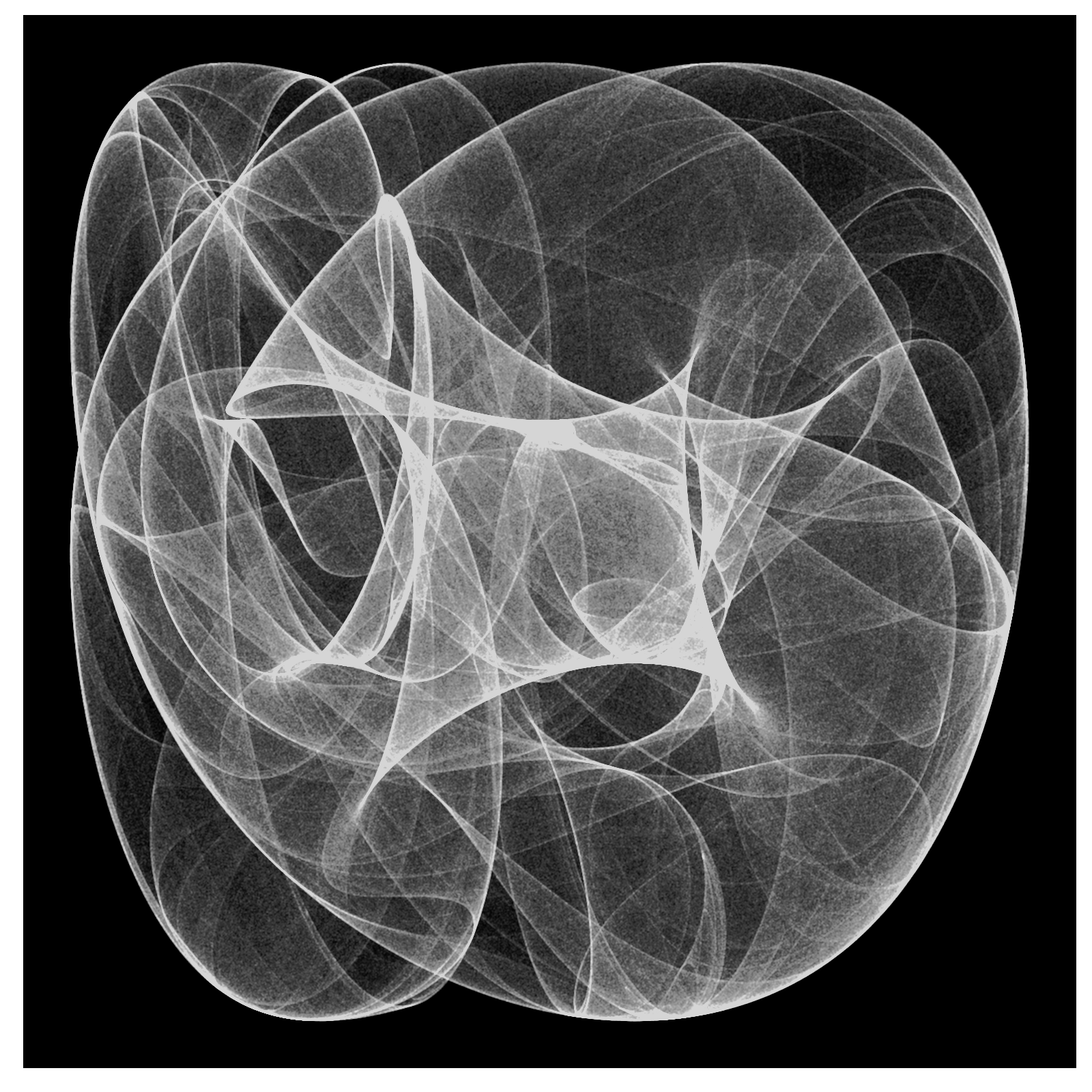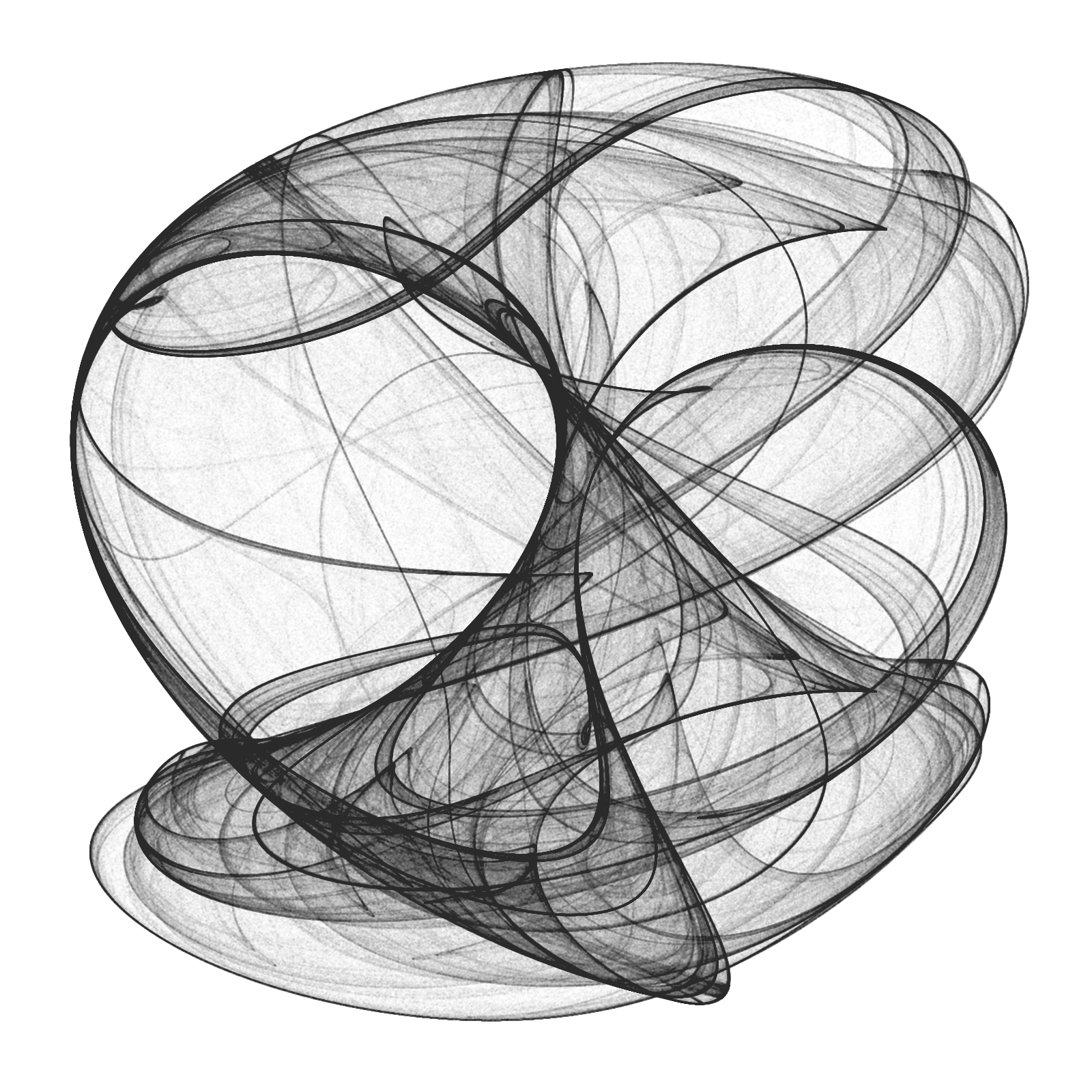From a technical point of view, the challenge is creating a data frame with all locations, since it must have 10 milion rows and must be populated sequentially. A very fast way to do it is using `Rcpp` package. To render the plot I use ggplot, which works quite well. Here you have the code to play with Clifford Attractors if you want:

```library(Rcpp)
library(ggplot2)
library(dplyr)

opt = theme(legend.position  = "none",
panel.background = element_rect(fill="white"),
axis.ticks       = element_blank(),
panel.grid       = element_blank(),
axis.title       = element_blank(),
axis.text        = element_blank())

cppFunction('DataFrame createTrajectory(int n, double x0, double y0,
double a, double b, double c, double d) {
// create the columns
NumericVector x(n);
NumericVector y(n);
x=x0;
y=y0;
for(int i = 1; i < n; ++i) {
x[i] = sin(a*y[i-1])+c*cos(a*x[i-1]);
y[i] = sin(b*x[i-1])+d*cos(b*y[i-1]);
}
// return a new data frame
return DataFrame::create(_["x"]= x, _["y"]= y);
}
')

a=-1.24458046630025
b=-1.25191834103316
c=-1.81590817030519
d=-1.90866735205054

df=createTrajectory(10000000, 0, 0, a, b, c, d)

png("Clifford.png", units="px", width=1600, height=1600, res=300)
ggplot(df, aes(x, y)) + geom_point(color="black", shape=46, alpha=.01) + opt
dev.off()
```

# Plants

Blue dragonflies dart to and fro
I tie my life to your balloon and let it go
(Warm Foothills, Alt-J)

In my last post I did some drawings based on L-Systems. These drawings are done sequentially. At any step, the state of the drawing can be described by the position (coordinates) and the orientation of the pencil. In that case I only used two kind of operators: drawing a straight line and turning a constant angle. Today I used two more symbols to do stack operations:

• “[“ Push the current state (position and orientation) of the pencil onto a pushdown
operations stack
• “]” Pop a state from the stack and make it the current state of the pencil (no line is drawn)

These operators allow to return to a previous state to continue drawing from there. Using them you can draw plants like these: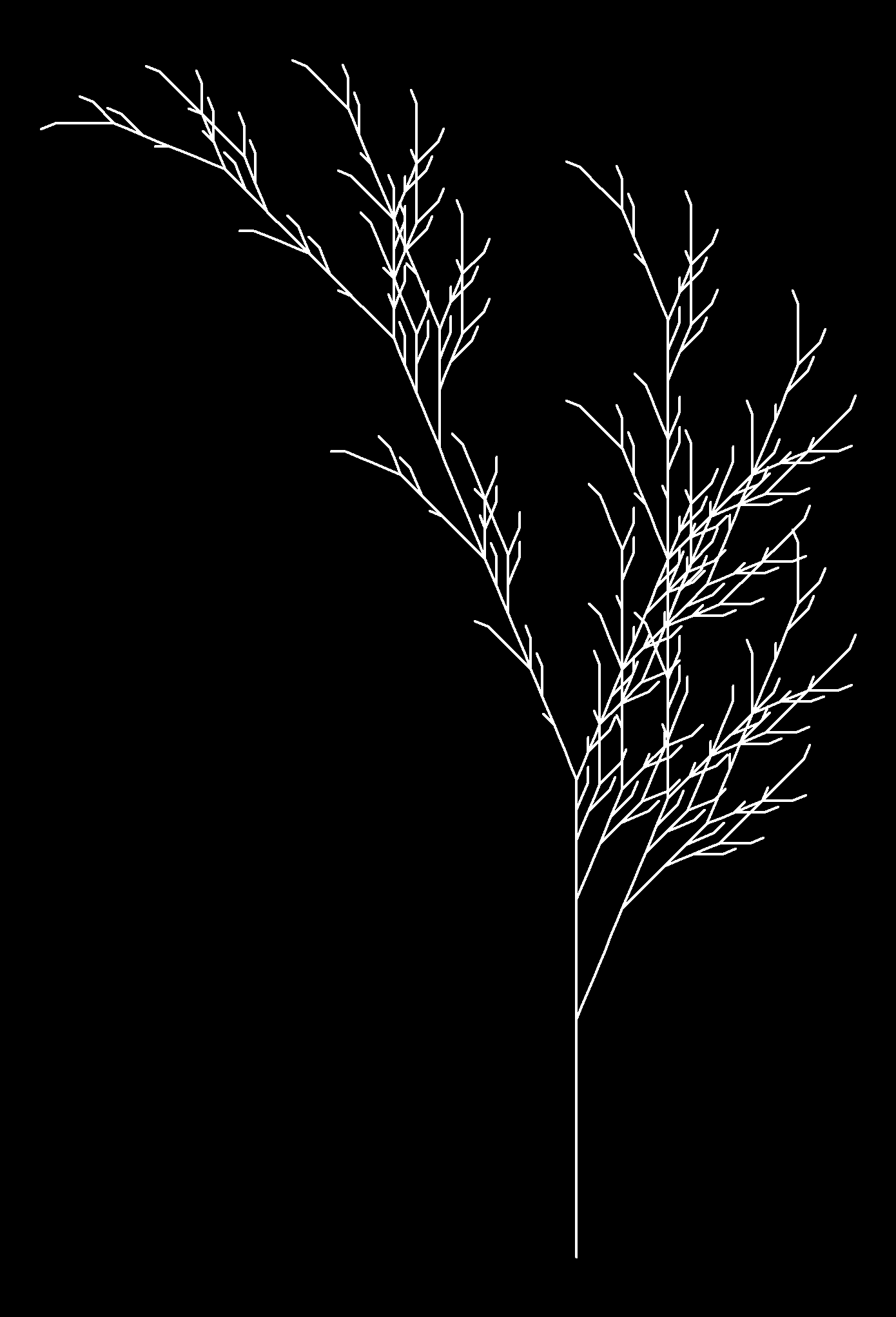Each image corresponds to a different axiom, rules, angle and depth. I described these terms in my previous post. If you want to reproduce them you can find the code below (each image corresponds to a different set of axiom, rules, angle and depth parameters). Change colors, add noise to angles, try your own plants … I am sure you will find nice images:

```
library(gsubfn)
library(stringr)
library(dplyr)
library(ggplot2)

#Plant 1
axiom="F"
rules=list("F"="FF-[-F+F+F]+[+F-F-F]")
angle=22.5
depth=4

#Plant 2
axiom="X"
rules=list("X"="F[+X][-X]FX", "F"="FF")
angle=25.7
depth=7

#Plant 3
axiom="X"
rules=list("X"="F[+X]F[-X]+X", "F"="FF")
angle=20
depth=7

#Plant 4
axiom="X"
rules=list("X"="F-[[X]+X]+F[+FX]-X", "F"="FF")
angle=22.5
depth=5

#Plant 5
axiom="F"
rules=list("F"="F[+F]F[-F]F")
angle=25.7
depth=5

#Plant 6
axiom="F"
rules=list("F"="F[+F]F[-F][F]")
angle=20
depth=5

for (i in 1:depth) axiom=gsubfn(".", rules, axiom)

actions=str_extract_all(axiom, "\\d*\\+|\\d*\\-|F|L|R|\\[|\\]|\\|") %>% unlist

status=data.frame(x=numeric(0), y=numeric(0), alfa=numeric(0))
points=data.frame(x1 = 0, y1 = 0, x2 = NA, y2 = NA, alfa=90, depth=1)

for (action in actions)
{
if (action=="F")
{
x=points[1, "x1"]+cos(points[1, "alfa"]*(pi/180))
y=points[1, "y1"]+sin(points[1, "alfa"]*(pi/180))
points[1,"x2"]=x
points[1,"y2"]=y
data.frame(x1 = x, y1 = y, x2 = NA, y2 = NA,
alfa=points[1, "alfa"],
depth=points[1,"depth"]) %>% rbind(points)->points
}
if (action %in% c("+", "-")){
alfa=points[1, "alfa"]
points[1, "alfa"]=eval(parse(text=paste0("alfa",action, angle)))
}
if(action=="["){
data.frame(x=points[1, "x1"], y=points[1, "y1"], alfa=points[1, "alfa"]) %>%
rbind(status) -> status
points[1, "depth"]=points[1, "depth"]+1
}

if(action=="]"){
depth=points[1, "depth"]
points[-1,]->points
data.frame(x1=status[1, "x"], y1=status[1, "y"], x2=NA, y2=NA,
alfa=status[1, "alfa"],
depth=depth-1) %>%
rbind(points) -> points
status[-1,]->status
}
}

ggplot() +
geom_segment(aes(x = x1, y = y1, xend = x2, yend = y2),
lineend = "round",
colour="white",
data=na.omit(points)) +
coord_fixed(ratio = 1) +
theme(legend.position="none",
panel.background = element_rect(fill="black"),
panel.grid=element_blank(),
axis.ticks=element_blank(),
axis.title=element_blank(),
axis.text=element_blank())
```

# A Shiny App to Draw Curves Based on L-System

Don’t worry about a thing ’cause every little thing gonna be alright (Three Little Birds, Bob Marley)

One of my favourite books is The Computational Beauty of Nature by Gary William Flake where there is a fantastic chapter about fractals in which I discovered the L-Systems.

L-Systems were conceived  in 1968 by Aristide Lindenmayer, a Hungarian biologist, as a mathematical description of plant growth. Apart from the Wikipedia, there are many places on the Internet where you can read about them. If you are interested, don’t miss The Algorithmic Beauty of Plants, an awesome book by Przemysław Prusinkiewicz that you can obtain here for free.

Roughly speaking, a L-System is a very efficient way to make drawings. In its simplest way consists in two different actions: draw a straigh line and change the angle. This is just what you need, for example, to draw a square: draw a straigh line of  any length, turn 90 degrees (without drawing), draw another straigh line of the same length, turn 90 degrees in the same direction, draw, turn and draw again. Denoting `F` as the action of drawing a line of length d and `+` as turning 90 degrees right, the whole process to draw a square can be represented as `F+F+F+F`.

L-Systems are quite simple to program in R. You only need to substitute the rules iteratively into the axiom (I use `gsubfn` function to do it) and split the resulting chain into parts with `str_extract_all`, for example. The result is a set of very simple actions (draw or turn) that can be visualized with `ggplot` and its path geometry. There are four important parameters in L-Systems:

• The seed of the drawing, called axiom
• The substitutions to be applied iteratively, called rules
• How many times to apply substitutions, called depth
• Angle of each turning

For example, let’s define the next L-System:

• Axiom: `F-F-F-F`
• Rule: `F → F−F+F+FF−F−F+F`

The rule means that every `F` must be replaced by `F−F+F+FF−F−F+F` while `+` means right turning and `-` left one. After one iteration, the axiom is replaced by `F-F+F+FF-F-F+F-F-F+F+FF-F-F+F-F-F+F+FF-F-F+F-F-F+F+FF-F-F+F` and iterating again, the new string is `F-F+F+FF-F-F+F-F-F+F+FF-F-F+F+F-F+F+FF-F-F+F+F-F+F+FF-F-F+FF-F+F+FF-F-F+F-F-F+F+FF-F-F+F-F-F+F+FF-F-F+F+F-F+F+FF-F-F+F-F-F+F+FF-F-F+F-F-F+F+FF-F-F+F+F-F+F+FF-F-F+F+F-F+F+FF-F-F+FF-F+F+FF-F-F+F-F-F+F+FF-F-F+F-F-F+F+FF-F-F+F+F-F+F+FF-F-F+F-F-F+F+FF-F-F+F-F-F+F+FF-F-F+F+F-F+F+FF-F-F+F+F-F+F+FF-F-F+FF-F+F+FF-F-F+F-F-F+F+FF-F-F+F-F-F+F+FF-F-F+F+F-F+F+FF-F-F+F-F-F+F+FF-F-F+F-F-F+F+FF-F-F+F+F-F+F+FF-F-F+F+F-F+F+FF-F-F+FF-F+F+FF-F-F+F-F-F+F+FF-F-F+F-F-F+F+FF-F-F+F+F-F+F+FF-F-F+F`. As you can see, the length of the string grows exponentially. Converting last string into actions, produces this drawing, called Koch Island:It is funny how different axioms and rules produce very different drawings. I have done a Shiny App to play with L-systems. Although it is quite simple, it has two interesting features I would like to undeline:

• Delay reactions with `eventReactive` to allow to set depth and angle values before refreshing the plot
• Build a dynamic UI that reacts to user input depending on the curve choosen

There are twelve curves in the application: Koch Island (and 6 variations), cuadratic snowflake, Sierpinsky triangle, hexagonal Gosper, quadratic Gosper and Dragon curve. These are their plots:

The definition of all these curves (axiom and rules) can be found in the first chapter of the Prusinkiewicz’s book. The magic comes when you modify angles and colors. These are some examples among the infinite number of possibilities that can be created:

I enjoyed a lot doing and playing with the app. You can try it here. If you do a nice drawing, please let me know in Twitter or dropping me an email. This is the code of the App:

`ui.R`:

```library(shiny)

shinyUI(fluidPage(
titlePanel("Curves based on L-systems"),

sidebarLayout(
sidebarPanel(
selectInput("cur", "Choose a curve:",
c("","Koch Island",
"Koch Variation 1",
"Koch Variation 2",
"Koch Variation 3",
"Koch Variation 4",
"Koch Variation 5",
"Koch Variation 6",
"Sierpinsky Triangle",
"Dragon Curve",
"Hexagonal Gosper Curve",
selected = ""),

conditionalPanel(
condition = "input.cur != \"\"",
uiOutput("Iterations")),

conditionalPanel(
condition = "input.cur != \"\"",
uiOutput("Angle")),

conditionalPanel(
condition = "input.cur != \"\"",
selectInput("lic", label = "Line color:", choices = colors(), selected = "black")),

conditionalPanel(
condition = "input.cur != \"\"",
selectInput("bac", label = "Background color:", choices = colors(), selected = "white")),

conditionalPanel(
condition = "input.cur != \"\"",
actionButton(inputId = "go", label = "Go!",
style="color: #fff; background-color: #337ab7; border-color: #2e6da4"))

),
mainPanel(plotOutput("curve", height="550px", width = "100%"))
)

))
```

`server.R`:

```library(shiny)
library(gsubfn)
library(stringr)
library(dplyr)
library(ggplot2)
library(rlist)

shinyServer(function(input, output) {

curves=list(
list(name="Koch Island",
axiom="F-F-F-F",
rules=list("F"="F-F+F+FF-F-F+F"),
angle=90,
n=2,
alfa0=90),
axiom="-F",
rules=list("F"="F+F-F-F+F"),
angle=90,
n=4,
alfa0=90),
list(name="Koch Variation 1",
axiom="F-F-F-F",
rules=list("F"="FF-F-F-F-F-F+F"),
angle=90,
n=3,
alfa0=90),
list(name="Koch Variation 2",
axiom="F-F-F-F",
rules=list("F"="FF-F-F-F-FF"),
angle=90,
n=4,
alfa0=90),
list(name="Koch Variation 3",
axiom="F-F-F-F",
rules=list("F"="FF-F+F-F-FF"),
angle=90,
n=3,
alfa0=90),
list(name="Koch Variation 4",
axiom="F-F-F-F",
rules=list("F"="FF-F--F-F"),
angle=90,
n=4,
alfa0=90),
list(name="Koch Variation 5",
axiom="F-F-F-F",
rules=list("F"="F-FF--F-F"),
angle=90,
n=5,
alfa0=90),
list(name="Koch Variation 6",
axiom="F-F-F-F",
rules=list("F"="F-F+F-F-F"),
angle=90,
n=4,
alfa0=90),
list(name="Sierpinsky Triangle",
axiom="R",
rules=list("L"="R+L+R", "R"="L-R-L"),
angle=60,
n=6,
alfa0=0),
list(name="Dragon Curve",
axiom="L",
rules=list("L"="L+R+", "R"="-L-R"),
angle=90,
n=10,
alfa0=90),
list(name="Hexagonal Gosper Curve",
axiom="L",
rules=list("L"="L+R++R-L--LL-R+", "R"="-L+RR++R+L--L-R"),
angle=60,
n=4,
alfa0=60),
axiom="-R",
rules=list("L"="LL-R-R+L+L-R-RL+R+LLR-L+R+LL+R-LR-R-L+L+RR-",
"R"="+LL-R-R+L+LR+L-RR-L-R+LRR-L-RL+L+R-R-L+L+RR"),
angle=90,
n=2,
alfa0=90))

output\$Iterations <- renderUI({ if (input\$cur!="") curve=list.filter(curves, name==input\$cur) else curve=list.filter(curves, name=="Koch Island") iterations=list.select(curve, n) %>% unlist
numericInput("ite", "Depth:", iterations, min = 1, max = (iterations+2))
})

output\$Angle <- renderUI({ curve=list.filter(curves, name==input\$cur) angle=list.select(curve, angle) %>% unlist
numericInput("ang", "Angle:", angle, min = 0, max = 360)
})

data <- eventReactive(input\$go, { curve=list.filter(curves, name==input\$cur) axiom=list.select(curve, axiom) %>% unlist
rules=list.select(curve, rules)[]\$rules
alfa0=list.select(curve, alfa0) %>% unlist

for (i in 1:input\$ite) axiom=gsubfn(".", rules, axiom)
actions=str_extract_all(axiom, "\\d*\\+|\\d*\\-|F|L|R|\\[|\\]|\\|") %>% unlist

points=data.frame(x=0, y=0, alfa=alfa0)
for (i in 1:length(actions))
{
if (actions[i]=="F"|actions[i]=="L"|actions[i]=="R")
{
x=points[nrow(points), "x"]+cos(points[nrow(points), "alfa"]*(pi/180))
y=points[nrow(points), "y"]+sin(points[nrow(points), "alfa"]*(pi/180))
alfa=points[nrow(points), "alfa"]
points %>% rbind(data.frame(x=x, y=y, alfa=alfa)) -> points
}
else{
alfa=points[nrow(points), "alfa"]
points[nrow(points), "alfa"]=eval(parse(text=paste0("alfa",actions[i], input\$ang)))
}
}
return(points)
})

output\$curve <- renderPlot({
ggplot(data(), aes(x, y)) +
geom_path(color=input\$lic) +
coord_fixed(ratio = 1) +
theme(legend.position="none",
panel.background = element_rect(fill=input\$bac),
panel.grid=element_blank(),
axis.ticks=element_blank(),
axis.title=element_blank(),
axis.text=element_blank())
})

})
```